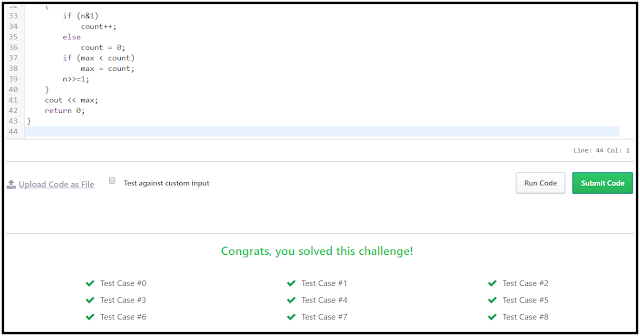# Day 10 Binary Numbers Hackerrank Solution in C & C++ | 30 Days

Find the Maximum Binary Sum Hackerrank Solution in C and C++. Write a Program to Day 10 Binary Numbers Hackerrank Solution in C & C++. The binary Numbers solution is divided into two parts one is a binary number and the second is consecutive 1's. In the problem, we can see that we have to find the maximum number of consecutive 1's. in a Number. This means of a consecutive number is continuing the same number to repeat maximum times. Below is the explanation of consecutive 1's with an example.

Explanation

For this problem, we are taking some numbers and also finding the binary of all numbers after that we can find the maximum consecutive 1's, take an example with a number between 1 to 15 and also write their binary number.

As we can see the maximum consecutive 1's in binary numbers are {15(4 consecutive 1's), (14, 7(consecutive 1's)), (3, 6, 11, 12, 13(consecutive 1's)), (1, 2, 4, 5, 8, 9, 10(1 consecutive 1's)), 0 (0 consecutive 1's). And here n & 1 produces a value that is either 1 or 0, depending on the least significant bit. This is a bitwise AND operation. or n >>= 1 means set n to itself shifted by one bit to the right. The expression evaluates to the new value of x after the shift.

Note: We can see that maximum consecutive 1's and maximum consecutive 0's may be less and equal to binary number digit(maximum digit in binary number).

All solution provided here are in C++ (CPP) if any reader wants these solutions in C, and Java comments below or sends a mail with your query like " day n solution in C / C++ / Java. Check the end of the post solutions with the full explanation.

Recently Updated Post

## Maximum Binary Sum Hackerrank Solution in C

``````#include <math.h>

#include <stdio.h>

#include <string.h>

#include <stdlib.h>

#include <assert.h>

#include <limits.h>

#include <stdbool.h>

int main() {
int n, count = 0, max = 0;
scanf("%d", & n);

while (n) {
if (n & 1)
count++;
else
count = 0;
if (max < count)
max = count;
n >>= 1;
}
printf("%d", max);
return 0;
}``````

### Binary Numbers Hackerrank Solution in C++

``````#include <map>

#include <set>

#include <list>

#include <cmath>

#include <ctime>

#include <deque>

#include <queue>

#include <stack>

#include <string>

#include <bitset>

#include <cstdio>

#include <limits>

#include <vector>

#include <climits>

#include <cstring>

#include <cstdlib>

#include <fstream>

#include <numeric>

#include <sstream>

#include <iostream>

#include <algorithm>

#include <unordered_map>

using namespace std;

int main() {
int n, count = 0, max = 0;
cin >> n;
while (n) {
if (n & 1)
count++;
else
count = 0;
if (max < count)
max = count;
n >>= 1;
}
cout << max;
return 0;
}``````

Maximum Binary Sum Hackerrank Solution Output### Similar to Binary Numbers 30 Days of Code

• Hackerrank Solution For Day 11: 2D Arrays
• Day 12: Inheritance Hackerrank Solution
• Hackerrank Solution For Day 13: Abstract Classes
• Day 14: Scope Hackerrank Solution
• Hackerrank Solution For Day 15: Linked List
Previous Post
Next Post

#### post written by: Ghanendra Yadav

Hi, I’m Ghanendra Yadav, SEO Expert, Professional Blogger, Programmer, and UI Developer. Get a Solution of More Than 500+ Programming Problems, and Practice All Programs in C, C++, and Java Languages. Get a Competitive Website Solution also Ie. Hackerrank Solutions and Geeksforgeeks Solutions. If You Are Interested to Learn a C Programming Language and You Don't Have Experience in Any Programming, You Should Start with a C Programming Language, Read: List of Format Specifiers in C.